# KSEEB Class 10 Science Important Questions Chapter 5 Periodic Classification of Elements

Students can download Class 10 Science Chapter 5 Periodic Classification of Elements Important Questions, KSEEB SSLC Class 10 Science Important Questions and Answers helps you to revise the complete Karnataka State Board Syllabus and score more marks in your examinations.

## Karnataka SSLC Class 10 Science Important Questions Chapter 5 Periodic Classification of Elements

Question 1
What are chemical elements? Give four examples.
Chemical substances that are made up of only one kind of atoms are known as chemical elements. eg: Sodium, iron, silver, boron, helium etc.Question 2.
How many elements are known till date? How many of these elements are naturally occurring?
About 118 elements are known till date. Of these, 98 are naturally occurring chemical elements.

Question 3.
What was the need for classification of chemical elements? What are the advantages of classification?
During the 17th century only a few elements were known. As more and more chemical elements were discovered, the number of elements in the list of known chemical elements went on increasing.

It was thought necessary to classify the elements in some useful way so that their study would become easy and systematic. In the absence of a suitable way of classification, the study of the elements would have become difficult.

Classification of elements has the following advantages:

• Classification enables systematic study of chemical elements and their properties.
• It enables grouping together of elements with similar properties.
• It also enables us to know the different chemical compounds formed by different elements.

Question 4.
Which was the earliest attempt to classify chemical elements?
The earliest attempt to classify the elements resulted in grouping the then known elements as metals and non-metals.

Question 5.
What were the disadvantages of classifying chemical elements as metals and non-metals?
In the classification of elements into metals and non-metals, there was no justification to put least active metals and most active metals in one group. This was true of non-metals also. For instance, sodium is a highly active metal while gold is an inactive metal.

Placing them in a single group had no justification. Secondly, there were some chemical elements which had the properties of both metals and non-metals. The placing of these metals posed a problem.

Question 6.
Who was the first to classify chemical elements on the basis of their atomic mass?
Dobereiner was the first scientist to attempt the classification of chemical elements on the basis of their atomic mass.

Question 7.
What was the basis on which Dobereiner classified chemical elements?
Dobereiner classified chemical elements in groups of three in the increasing order of their atomic masses such that the atomic mass of the middle element is the average of the first and the third elements.

Question 8.
Define atomic mass of an element.
Atomic mass of an element is defined as the ratio between the mass of one atom of that element and one-twelfth the mass of one carbon-12 atom. It is also called relative atomic mass.Question 9.
According to Dobereiner’s law of triads, ‘when the elements are arranged in groups of three in increasing order of atomic masses, the atomic mass of the middle element is roughly the average of the atomic masses of the other two elements’.

Question 10.
Give two examples for Dobereiner’s law of triads.Question 11.
Calcium, strontium and barium taken in that order constitute Dobereiner’s triads. If the atomic masses of calcium and barium are 40 and 137 respectively, calculate the atomic mass of strontium.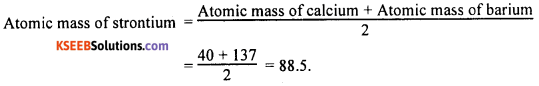Question 12.
Given below are some groups of three elements. These elements are arranged downwards in order of increasing atomic masses. find out which of these groups form dobereiner triads.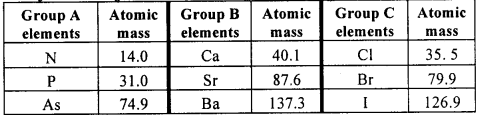Group A elements do not form Dobereiner triad as the average atomic mass of N and As is not equal to the atomic mass of P. Group B elements form Dobereiner triad as the average atomic mass of Ca and Ba is nearly equal to the atomic mass of Sr and these elements have similar properties.

Group C elements also form Dobereiner triad as the average atomic mass of Cl and I is more or less equal to the atomic mass of the middle element Br and these elements have similar properties.

Question 13.
A, B and C are elements of a Dobereiner’s triad. If the atomic mass of A is 7 and that of C is 39, calculate the atomic mass of B.
B is the middle element of the triad. Therefore,Question 14.
The atomic masses of sodium, silicon and chlorine are 22.9897, 28.0855 and 35.453 respectively. Do these elements constitute Dobereiner’s triads? Explain.
According to Dobereiner’s law, three elements arranged in the increasing order of atomic masses will constitute a triad if the average atomic mass of the extreme elements of the triad is equal to the atomic mass of the middle element.
Let us now find out the average of atomic masses of sodium and chlorine.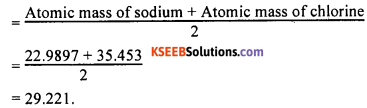The value 29.221 is nearly equal to the given value of atomic mass of silicon, which is 28.0855. However, they do not constitute Dobereiner’s triads, as they are dissimilar in their properties.

Question 15.
What were the limitations of Dobereiner’s classification?

OR

The classification of elements into triads was not found suitable for the following reasons: All known elements could not be classified into groups of triads on the basis of their properties. Secondly, not all groups obeyed the Law of Triads.

For example, nitrogen family does not obey the Law of Triads. Thirdly, Dobereiner could identify only three triads from the elements known at that time.

Question 16.
State Newland’s law of octaves. On what basis did Newland classify the chemical elements?
Newland’s law of octaves states that, ‘when elements are arranged in the increasing order of atomic masses, the properties of the eighth element are a kind of repetition of the first, just like the notes of music.’ Newland arranged the elements in the order of increasing atomic masses.

Question 17.
What is meant by periodicity ofproperties of chemical elements?
It was found that the properties of chemical elements repeated after a definite interval when the chemical elements were arranged in a certain order. This repetition of similar properties of chemical elements after a definite interval is known as periodicity.

Question 18.
Explain the periodicity found in Newland’s classification of elements with an example.
Newland’s Octaves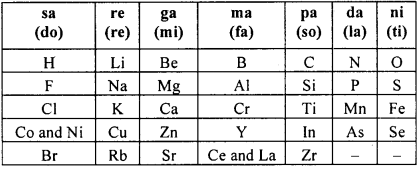Newland observed that when elements were arranged in the increasing order of their atomic masses, every eighth element had similar properties. Therefore, he placed the eighth element below the first, the ninth element below the second, the tenth element below the third, and so on.

For instance, he placed fluorine and chlorine below hydrogen. He placed sodium and potassium below lithium and so on.

Question 19.
A part of early classification of elements is shown below: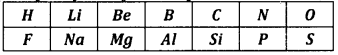1. Which law of classification is applicable here?
2. Who proposed this system of classification?
3. Why is such a classification compared with the notes on musical scale?
4. State one limitation of this type of classification of elements.

1. The elements above are classified on the basis of the law of octaves.
2. Newlands proposed this system of classification.
3. In this classification, the repetition of the properties of elements is just like the repetition of the eighth note in the octave music (sa, re, ga, ma, pa, da, ni, sa). This is why the classification is compared with the notes on musical scale.
4. This periodicity in properties could not be applied to all elements and it could only be applied up to calcium.Question 20.
What were the limitations of Newland”s Law of Octaves?
The limitations of Newlands’ Law of Octaves were:

1. It was found that the Law of Octaves was applicable only up to calcium. After calcium, the eighth element did not possess properties similar to that of the first.
2. Newlands assumed that only 56 elements existed in nature and no more elements would be discovered in future.
3. To fit the elements into his table, Newlands not only adjusted two elements in the same slot but also put some unlike elements under the same note.

Question 21.
Up to which element was the law of octaves found to be applicable?
The Law of Octaves was applicable up to calcium.

Question 22.
In order to fit elements into his table, Newlands adjusted two elements in the same slot, but also put some unlike elements under the same note. Give examples of these arrangements made by Newlands.
Newlands placed cobalt and nickel in the same slot and placed them in the same column as fluorine, chlorine and bromine, which have very different properties than these elements. Iron, which resembles cobalt and nickel in its properties, was placed far away from these elements.

Question 23.
What were the strengths of Newlands’ classification of elements?
Newlands’ Law of Octaves provided a basis for classification as it grouped elements with similar properties. It was for the first time that elements were classified on some logical basis (atomic mass). It paved the way for improvement in the way of classifying elements.

Question 24.
Did Dobereiner’s triads also exist in the columns of Newlands’ Octaves? Compare and find out.
Yes, the following triads can be identified in the columns of Newlands’ octaves:

• Li, Na, K
• Be, Mg, Ca.

The atomic mass of the middle element in the above triads is approximately equal to the average mass of the elements on the left and right hand sides.

Question 25.
State Mendeleev’s periodic law.
Mendeleev’s periodic law states that ‘the properties of elements are the periodic function of their atomic masses’.

Question 26.
What is a Periodic Table of elements?
A Periodic Table of elements is a chart of elements prepared in such a way that the elements having similar properties occur in the same vertical column i.e., same group.

Question 27.
Which two criteria did Mendeleev use to classify elements in his Periodic Table?
Mendeleev used the following two criteria to classify elements Atomic mass and similarity in chemical property of elements.

Question 28.
How did Mendeleev classify chemical elements? Explain.
During the time of Mendeleev, only 63 elements were known. He arranged these elements in the increasing order of their atomic masses. He found that the properties of elements showed periodicity at regular intervals. He found that every eighth element had properties similar to that of the first.

He used this periodicity to arrange the elements in periods and groups in the table. He left some gaps in the Periodic Table and indicated that some elements that should take these positions are yet to be discovered.

Question 29.
List the main features of Mendeleev’s Periodic Table.
The main features of Mendeleev’s Periodic Table are listed below

• The horizontal rows present in the Periodic Table are called periods. There are seven periods in the table.
• Properties of elements in a particular period show regular gradation from left to right.
• The vertical columns present in it are called groups. These are nine in number and are numbered from 1 to VIII and Zero.
• Groups I to VII are subdivided into A and B subgroups. Groups Zero and VIII do not have any subgroups.
• All the elements in a particular group are chemically similar in nature. They show regular gradation in their physical properties and chemical reactivity.

Question 30.
What are Periods and Groups in a Periodic Table?
The vertical columns of the Periodic Table are called ‘groups’. The horizontal rows of the Periodic Table are called ‘periods’.

Question 31.
List the advantages of Mendeleev’s Periodic Table.
Mendeleev could classify all the known elements at that time in his Periodic Table. He predicted the existence of many other elements and he could also predict their properties even before their discovery.

The elements he predicted were later discovered and had properties which Mendeleev had predicted. His Periodic Table helped to correct the atomic masses of certain elements on the basis of their position in the Periodic Table.

Question 32.
Name two elements predicted by Mendeleev much before their actual discovery.
Mendeleev had predicted a new element with atomic mass 68. He called this element Eka- aluminium. Later a new element called Gallium was discovered and its atomic mass turned out to be 69.7.

He had also predicted another element called Eka-silicon and stated its atomic mass to be 72. When this element was actually discovered, its atomic mass was found to be 72.59. This element is called Germanium.Question 33.
Name the elements whose existence was predicted by Mendeleev while designing his Periodic Table.
The elements predicted by Mendeleev and which were discovered later are

• Eka-boron (Scandium)
• Eka-aluminium (Gallium)
• Eka-silicon (Germanium).

Question 34.
Use Mendeleev’s Periodic Table to predict the formulae for the oxides of the following elements: K, C, Al, Si, Ba.
On the basis of Mendeleev’s Periodic Table, the formulae for the oxides of potassium (K), carbon (C), aluminium (Al), silicon (Si) and barium (Ba) are K2O, CO2, Al2O3, SiO2, and BaO respectively.

Question 35.
Besides gallium, which other elements have since been discovered that were left by Mendeleev in his Periodic Table? (Write any two.)
Besides gallium, two other elements that were left out by Mendeleev in his Periodic Table were scandium and germanium.

Question 36.
What were the criteria used by Mendeleev in creatine his Periodic Table?
Mendeleev created his Periodic Table on the basis of their fundamental property

• the atomic masses in increasing order
• on the similarity of chemical properties.

Among chemical properties he concentrated on the compounds formed by elements with oxygen and hydrogen.

Question 37.
Why do you think the noble eases are placed in a separate group?
Noble gases are inert elements. Their properties are different from all other elements. Therefore, the noble gases are placed in a separate group.

Question 38.
Why did Mendeleev leave gaps in his Periodic Table? Give an example to illustrate your answer.
During Mendeleev’s time many chemical elements were unknown. He could not fit any known element in some spaces in his Periodic Table. Instead of considering this as a defect, he boldly predicted the existence of those elements. He was sure that these elements would be discovered in future and even gave those elements a name.

For example, when Mendeleev created his table, inert gases didn’t exist but once they were discovered they could easily be accommodated in the spaces left by Mendeleev.

Question 39.
Did Mendeleev believe that there could be undiscovered elements? If so, how did he accommodate these elements in his Periodic Table?
Mendeleev predicted the existence of a few elements although they were not known to exist. While arranging the elements in the increasing order of their atomic masses, there were some spaces which could not be filled in by the existing elements. He left such spaces blank to accommodate those undiscovered elements.

Question 40.
How did Mendeleev name the elements whose existence he predicted?
Mendeleev named the elements he predicted by prefixing a Sanskrit numeral, Eka (one), to the name of preceding element in the same group. For instance, scandium, gallium and germanium, discovered later, have properties similar to Eka-boron, Eka-aluminium and Eka-silicon respectively.

The properties of Eka-aluminium predicted by Mendeleev were similar to that of the element gallium, which was discovered later. Thus gallium replaced Eka-aluminium in the Periodic Table.

Question 41.
Give a suitable evidence for both the correctness and usefulness of Mendeleev’s Periodic Table.
Mendeleev had predicted the existence of a few elements such as Eka-boron, Eka-aluminium and Eka-silicon. He even predicted the properties of these elements. Elements such as scandium, gallium and germanium, which were discovered later, have properties similar to Eka-boron, Eka-aluminium and Eka- silicon, respectively. As an example, the properties of Eka-aluminium predicted by Mendeleev and those of gallium are listed in the table belowThis is an evidence for both the correctness and usefulness of Mendeleev’s Periodic Table.

Question 42.
Looking at its resemblance to alkali metals and the halogen family, try to assign a correct position for hydrogen in Mendeleev’s Periodic Table.
The properties of hydrogen are similar to those of both alkali metals and halogens. The electronic configuration of hydrogen resembles that of alkali metals. Both alkali metals and hydrogen have just one electron in the valence shell. Hydrogen, just like alkali metals, combines with halogens, oxygen and sulphur to form compounds having similar formulae.

Therefore, there is a case for placing hydrogen in group I along with alkali metals. On the other hand, the properties of hydrogen resemble those of halogens. Both hydrogen and halogens exist as diatomic molecules. Both hydrogen and halogens combine with metals end non-metals to form covalent compounds.

Therefore, there is a case for placing hydrogen in group VII along with halogens. Thus it is difficult to assign with certainty a suitable position for hydrogen in Mendeleev’s Periodic Table. This is a limitation of Mendeleev’s Periodic Table.Question 43.
Chlorine has two isotopes namely Cl-35 and Cl-37. Where do you place these two in Mendeleev’s Periodic Table?
In Mendeleev’s Periodic Table, elements are arranged in the increasing order of their atomic masses. Therefore, the two isotopes of chlorine must be placed in two different positions in the table. But there is no vacant position available in his Periodic Table for placing both isotopes suitably. This is a limitation of Mendeleev’s classification of elements.

Question 44.
What are the limitations of Mendeleev’s Periodic Table?
The following are some of the limitations of Mendeleev’s Periodic Table:
1. Mendeleev could not assign a correct position for hydrogen in his Periodic Table, as the properties of hydrogen resemble that of both alkali metals as well as halogens.

2. The isotopes of the same element will have to be given different positions if atomic mass is taken as the basis. This would disturb the symmetry of the Periodic Table.

3. The atomic masses do not increase in a regular manner in going from one element to the next. So it was not possible to predict how many elements could be discovered between two elements.

Question 45.
Taking atomic masses of elements as the basis is not useful for constructing the Periodic Table. Give reasons with a suitable example.
Many of the chemical elements have their isotopes. The isotopes of an element have different masses. For example, carbon has three known isotopes namely C-12, C-13 and C-14. If elements are arranged in the Periodic Table on the basis of atomic mass, carbon should have three positions for its isotopes.

Similarly hydrogen has three isotopes. Therefore, three boxes are to be reserved for hydrogen in the Periodic Table. This is both inconvenient and cumbersome. Hence atomic mass is not useful for constructing a Periodic Table.

Question 46.
Who suggested that atomic number and not atomic mass is the fundamental property for classification of elements?
An English physicist by name Henry Moseley suggested that atomic number and not atomic mass is the fundamental property for classification of elements.

Question 47.
Define atomic number of an element.
Atomic number of an element is defined as the number of protons present in the nucleus of its atom. It is a unique number for a given element.

Question 48.
Why is atomic number more important in the classification of elements?

OR

Atomic number is considered to be a more appropriate parameter than atomic mass for classification of elements in a Periodic Table. Why?

OR

What is the advantage of using atomic number instead of atomic mass while constructing modern periodic table?
The chemical properties of elements depend on the number of valence electrons, which depend upon the atomic number. Hence atomic number is more important. Hence atomic number is a more appropriate parameter than atomic mass for the classification of elements.

Question 49.
State the modern periodic law.
Modem periodic law states that ‘the properties of the elements are periodic functions of their atomic numbers.’Question 50.
State two advantages of classifying elements on the basis of their atomic number.
All isotopes of an element have the same atomic number and their chemical properties are similar. Therefore, we can assign one and the same place to all the isotopes of an element. Secondly, prediction of properties of elements could be made with more precision when elements are arranged on the basis of increasing atomic number.

Question 51.
How are the elements arranged in the Modern Periodic Table?
In the Modern Periodic Table, the elements are arranged in the increasing order of their atomic numbers based on the modern periodic law, which states that ‘the properties of the elements are periodic functions of their atomic numbers’.

Question 52.
What are isotopes ? Give an example.
Atoms of the same element with different mass numbers are called isotopes. For example, carbon has three known isotopes. They are carbon-12, carbon-13 and carbon-14. All these atoms have the same atomic number and hence occupy the same place in the Modern Periodic Table.

Question 53.
Why are isotopes of an element given the same place in the Modern Periodic Table?
Isotopes of an element are atoms with same atomic number but different atomic mass. In the Modern Periodic Table, the elements are arranged in the increasing order of their atomic numbers in accordance with the modern periodic law. Since isotopes of an element have the same atomic number, they are given the same place in the Periodic Table.

Question 54.
How were the positions of cobalt and nickel resolved in the Modern Periodic Table?
In the Modern Periodic Table, elements are arranged in the increasing order of their atomic numbers. Atomic numbers of cobalt and nickel are 27 and 28 respectively. Therefore, cobalt is placed in group 9 and nickel is placed in group 10 in the Modern Periodic Table.

Question 55.
The atomic number of hydrogen is 1 and the atomic number of helium is 2. Is it possible to have an element with atomic number 1.5 placed between hydrogen and helium?
An element with atomic number 1.5 is not possible. This is because the atomic numbers of elements are always whole numbers.

Question 56.
Name all the elements present in group 1 of the Modern Periodic Table. Write down the electronic configuration of the first three elements. What similarity do you find in their electronic configurations? How many valence electrons are present in these three elements?
The elements present in group 1 of the Modern Periodic Table are hydrogen (H), lithium (Li), sodium (Na), potassium (K), rubidium (Rb), caesium (Cs) and francium (Fr). Thus, there are seven elements in group 1.
The electronic configurations of the First three elements are given below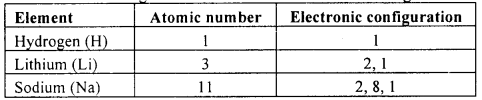All elements in group 1 have just one electron in the valence shell.

Question 57.
The elements Li, Be, B, C, N, O, F, and Ne are placed in the second period of the Modern Periodic Table. Write their electronic configurations. Do these elements contain the same number of valence electrons? Do they contain the same number of shells?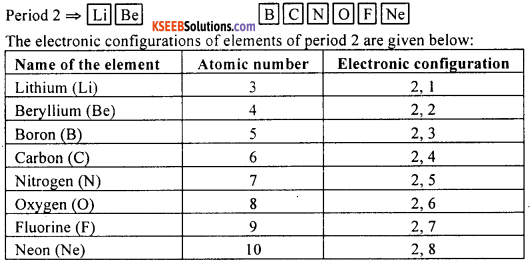No, the elements of the same period do not contain the same number of valence electrons. The number of valence electrons increases as we move in a period from left to right. Yes. All elements of the same period have the same number of filled shells. In period 2, there are two shells namely K and L.Question 58.
Elements such as Na, Mg, Al, Si, P, S, Cl and Ar belong to the third period of the Modern Periodic Table. Write their electronic configurations. Why are these elements placed in the same period?
The electronic configurations of the elements in period 3 are given below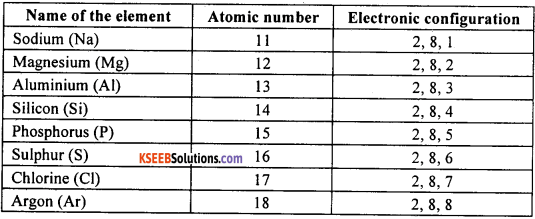The number of occupied shells in each of the elements above is 3 (K, L and M). Therefore all these elements are placed in period 3 of the Modem Periodic Table.

Question 59.
Name the element which has

1. two shells, both of which are completely filled with electrons
2. the electronic configuration 2, 8, 2
3. a total of three shells, with four electrons in its valence shell
4. a total of two shells, with three electrons in its valence shell
5. twice as many electrons in its second shell as in its first shell.

1. Neon
2. Magnesium
3. Silicon
4. Boron
5. Carbon.

Question 60.
Describe the main features of the Modern Periodic Table.
The main features of the Modem Periodic Table are:

1. The Modern Periodic Table consists of vertical columns called groups and horizontal rows called periods.
2. Elements are arranged in the increasing order of atomic numbers.
3. Elements in the Modem Periodic Table are arranged in 7 periods and 18 groups.
4. Elements are placed in periods based on the number of shells in their atoms. For example, all elements which have one occupied shell are placed in period 1, elements which have two occupied shells are placed in period 2 and so on.
5. The number of valence electrons increases by one as we move from left to right in a given period.
6. All elements in a given group contain the same number of valence electrons.
7. The number of occupied shells increases as we move from top to bottom in a given group.
8. The atomic radius decreases as we move from left to right along a period.
9. Metals are placed on the left hand side of the Periodic Table while non-metals occupy the right hand side. Metalloids are placed between metals and non-metals.
10. The noble gases with valency zero are placed in the 18th group.
11. The metallic character decreases as we move from left to right in a period.
12. The metallic character increases as we move from top to bottom in a group.

Question 61.
Observe the given table and answer the following question: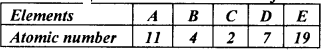Identify the two elements that belong to the same period and the two elements that belong to the same group. Give reason for your conclusion.
Among the elements given, elements B and D have electronic configuration 2, 2 and 2, 5 respectively. Both elements have two shells with electrons. Therefore they belong to the same period namely the second period.

Among the elements given, elements A (atomic number 11) and E (atomic number 19) have electronic configuration 2, 8, 1 and 2, 8, 7 respectively. The valence number of both A and E is same (equal to 1). Therefore, both A and E are in the same group.

Question 62.
How many elements are there in the first, second, third and fourth periods?
There are just two elements in the first period. The second and the third periods contain eight elements each. The fourth period contains 18 elements. Thus, the first period is the shortest period in the Modern Periodic Table.Question 63.
How could the Modern Periodic Table remove various anomalies of Mendeleev’s Periodic Table?
In the Modern Periodic Table, elements are arranged in the increasing order of their atomic numbers. This settled the placement of isotopes as isotopes have the same atomic number. The position of hydrogen is also settled in Modern Periodic Table.

Question 64.
Name two elements you would expect to show chemical reactions similar to that of magnesium. What is the basis for your choice?
The two elements expected to show chemical reactions similar to magnesium are calcium and strontium. This is because the number of valence electrons (2) is same in all these three elements. Since chemical properties are due to valence electrons, they show similar chemical reactions.

Question 65.
Name (a) three elements that have a single electron in their outermost shell, (b) two elements that have two electrons in their outermost shell, and (c) three elements with filled outermost shells.

• Lithium, sodium and potassium have a single electron in their outermost shell.
• Magnesium and calcium have two electrons in their outermost shell.
• Helium, neon and argon have completely filled outermost shells. Helium has two electrons in the valence shell whereas neon and argon have eight electrons each in their valence shell.

Question 66.

1.  Lithium, sodium, potassium are all metals that react with water to liberate hydrogen gas. Is there any similarity in the atoms of these elements?
2. Helium is an unreactive gas and neon is a eas of extremely low reactivity. What, if anything, do their atoms have in common?

1. Lithium, sodium and potassium have same number of electrons in the outermost shell.
2. Helium and neon have completed outermost shell, 2 electrons in the case of helium and 8 electrons in the case of neon.

Question 67.
Define the following terms: Atomic size, valence electrons, ionisation potential, electron affinity, metal, non-metal, metalloids.

• Atomic size: The radius of the atom is called its atomic size. It is the distance between the centre of the nucleus and the outermost shell of an isolated atom.
• Valence electrons: The number of electrons present in the outermost orbit of an atom is called valence electrons.
• Ionisation potential: The energy required to remove completely an electron from its atom is called ionisation potential.
• Electron affinity: The ability of an atom or molecule to form a negative ion is called electron affinity. It is expressed as the energy released when an electron is attached to it.
• Metal: A chemical element which is typically hard, shiny, malleable, fusible, and ductile, with good electrical and thermal conductivity is called a metal.
• Non-metal: A chemical element which is soft, neither malleable nor ductile and a poor conductor of heat and electricity is called a non-metal.
• Metalloids: Elements that have some properties of both metals and non-metals are called metalloids.Question 68.
Define valency of an element.
A measure of the combining capacity of an element with atoms of other elements while forming compounds is called valency.

Question 69.
How do you calculate the valency of an element from its electronic configuration?
The valency of an atom is equal to the number of electrons in the outermost shell if that number is 4 or less. Otherwise, the valency is equal to 8 minus the number of electrons in the outer shell. If the number of electrons are known, the valency can be calculated easily.

For elements of groups 1, 2, 13 and 14 the valency is equal to the number of valence electrons. For elements of groups 15 to 18, the valency is equal to  minus [No. of valence electrons].

Question 70.
How does the valency vary in a period as we move from left to right?
As we move from left to right in a given period of the Modern Periodic Table, the valency first increases from 1 to 4 and then decreases from 4 to zero.

Question 71.
How does the valency of elements vary as we go down a group?
In a given group, all elements have the same number of valence electrons. Therefore, all elements in a given group have the same valency. For example, all elements in group 2 have valency 2. All elements in group 13 have valency 3 and so on.

Question 72.
What is the valency of magnesium with atomic number 12 and sulphur with atomic number 16?
The electronic configuration of magnesium is 2, 8, 2. This element is in group 2. Therefore, the valency of magnesium is 2.
The electronic configuration of sulphur is 2, 8, 6. This element is in group 16. Therefore, the valency of sulphur is (8 – 6) = 2.

Question 73.
Explain with an example that the number of valence electrons in elements increases from 1 to 8 as we move from left to right in a period.
Let us consider the elements of the third period of the Modern Periodic Table and know their electronic configurationIn the table above, the number of valence electrons increases from 1 to 8 as we move from left to right across a period.

Question 74.
What is meant by atomic size of an element?
The radius of the atom of an element is called its atomic size. It is the distance between the centre of the nucleus and the outermost shell of an isolated atom.

Question 75.
How does the atomic radius change as we go (i) from left to right in a period, (ii) top to bottom in a group?
As we move from left to right in a period, the atomic radius decreases. As we go from top to bottom in a group, the atomic size increases.

Question 76.
Why does the atomic size decrease as we move from left to right in a period? Why does the atomic size increase as we move from top to bottom in a group?
Atomic size gradually decreases from left to right across a period of elements. This is because, as we move from left to right within a period, there is an increase in nuclear charge which tends to pull the electrons closer to the nucleus and reduces the size of the atom.

The atomic size increases as we move down from top to bottom in a group. This is because new shells are being added as we go down the group. This increases the distance between the outermost electrons and the nucleus so that the atomic size increases in spite of the increase in nuclear charge.Question 77.
Distinguish between metals and non-metals.
Metals:

1. All metals except mercury are solids at room temperature. Mercury is a liquid.
2. Metals are generally malleable and ductile.
3. Metals are good conductors of heat and electricity.
4. Metals are electro-positive in nature. They tend to lose electrons during chemical reactions.

Non-metals:

1. Non-metals occur in all the three states namely solid, liquid and gaseous states.
2. Non-metals are brittle. They are neither malleable nor ductile.
3. Non-metals are bad conductors of heat and electricity.
4. Most non-metals are electro-negative in nature. They tend to gain electrons during chemical reactions.

Question 78.
In the Modern Periodic Table, which are metals amons the first ten elements?
Among the first ten elements in the Modem Periodic Table, Lithium and Beryllium are metals.

Question 79.
List all the elements of the third period of the Modern Periodic Table. Classify them as metals and non-metals. On which side of the Periodic Table do you find metals? On which side of the Periodic Table do you find the non-metals?
The elements found in the third period of the Periodic Table areOf these elements, Na, Mg, and A1 are metals. P, S, Cl and Ar are non-metals. Si is a metalloid. Metals lie on the left side of the Periodic Table whereas non-metals lie on the right side of the Periodic Table.

Question 80.
In which part of the Periodic Table do we mostly find non-metals?
We find non-metals towards the right side of the Modern Periodic Table.

Question 81.
What are metalloids? Give examples. Where are these placed in the Periodic Table?
Chemical elements that have properties in between those of metals and non-metals are known as metalloids. eg: boron, silicon, germanium, arsenic, antimony, tellurium, polonium etc. Metalloids are placed in the middle of the Modern Periodic Table between metals and non-metals.

Question 82.
In the Modem Periodic Table, the element calcium (atomic number 20) is surrounded by elements with atomic numbers 12, 19, 21 and 38. W!ich of these have properties similar to that of calcium?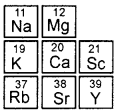In the Modern Periodic Table elements magnesium, calcium and strontium fall in the same group (group II). They have similar electronic configurations. All the three elements have 2 electrons in their outermost shell. Therefore the properties of calcium are similar to that of magnesium and strontium.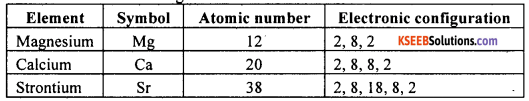Question 83.
Why should atoms give up or receive electrons? In which case do atoms hot receive or give up electrons but share them?
Atoms have a tendency to attain stable structure of noble gases (either duplet structure or octet structure) during chemical reactions. This usually occurs either by the

• transfer of electrons from one atom (donor atom) to another (receiver atom). The donor atom is electropositive while the receiver atom is electronegative. This happens in ionic compounds.
• sharing of electrons between two or more atoms. This happens in covalent compounds.

Question 84.
How does electropositive character and electronegative character of elements change along the periods and groups?
Electropositive nature (tendency to lose electrons) of elements decreases as we move from left to right along a period. Electronegative character (tendency to gain electrons) of elements increases as we move across a period.

The electropositive character of elements increases as we move down a group. The electronegative character of elements decreases as we move down a group.Question 85.
How would the tendency to gain electrons change as we move from left to right across a period?
As we move from left to right across a period, the nuclear charge increases regularly by one unit. So the force of attraction for the incoming electron increases. Therefore, the tendency to gain electron increases across a period.

Question 86.
How would the tendency to gain electrons change as we go down a group? Explain why.
The tendency to gain electrons decreases as we go down a group. As we go down a group, the atomic size progressively increases. As a result, the force of attraction of the nucleus for the incoming electron decreases. Therefore, the tendency to gain electrons decreases.

Question 87.
By considering their position in the Periodic Table, which one of the following elements would you expect to have maximum metallic characteristics: Ga, Ge, As, Se, and Be?
Metallic character of an element increases as we go down a group and decreases as we move from left to right in a period. On this basis Beryllium (Be) is expected to have the highest metallic character.

Question 88.

1. What property do all elements in the same column of the Periodic Table as boron have in common?
2. What property do all elements in the same column of the Periodic Table as fluorine have in common?

1. All the elements placed in the same column as boron have three electrons in the valence shell. This means all the elements in the column are trivalent.

2. All the elements placed in the same column along with fluorine have seven electrons in the valence shell. Therefore, their valency is one. This means all these elements are monovalent. All the elements are non-metals.

Question 89.
An atom has electronic configuration 2, 8, 7.

1. What is the atomic number of this element?
2. To which of the following elements would it be chemically similar.

(Atomic numbers are eiven in parentheses.) N(7), F(9), P(15), Ar(18)?
Chlorine has the electronic configuration 2, 8, 7.

1. Atomic number of the element is 17.
2. The element with electronic configuration 2, 8, 7 is chemically similar to fluorine (F) whose atomic number is 9.

Question 90.
The positions of three elements A, B and C in the Periodic Table are shown below

 Group 16 Group 17 – – – A – – B C
1. State whether A is a metal or non-metal.
2. State whether C is more reactive or less reactive than A.
3. Will C be larger or smaller in size than B?
4. Which type of ion, cation or anion, will be formed by element A?

1. A is a non-metal.
2. C is less reactive than A.
3. C will be smaller than B.
4. A will form anion.Question 91.
Nitrogen (atomic number 7) and phosphorus (atomic number 15) belong to Group 15 of the Periodic Table. Write the electronic configuration of these two elements. Which of these will be more electronegative? Why?
The atomic number of nitrogen is 7. Its atom has 7 electrons with electronic configuration 2, 5. The atomic number of phosphorus is 15. Its atom has 15 electrons with electronic configuration 2, 8, 5. Non-metallic character decreases as we move down a group. Therefore, nitrogen will be more electronegative than phosphorus.

Question 92.
How does the electronic configuration of an atom relate to its position in the Modern Periodic Table?
The electronic configuration of an atom gives the number of electrons in its outermost shell. Based on this, the group number of the element can be predicted. The number of valence electrons indicates the group to which it belongs.

The electronic configuration of an atom tells the number of shells with filled electrons. The number of shells with electrons is the period of that element.
This is how electronic configuration of an element and its position in the Modern Periodic Table are related.

Question 93.
Compare and contrast the arrangement of elements in Mendeleev’s Periodic Table and the Modern Periodic Table.
Mendeleev’s Periodic Table:

1. The elements are arranged in the increasing order of atomic masses.
2. The position of an element depends on its atomic mass and properties.
3. It is difficult to place isotopes as their masses are different. The position of the isotopes is not justified.
4. Arranging elements on the basis of atomic mass led to some anomalies.
5. Does not properly justify the periodicity of the properties of elements.
6. There is no clear-cut separation of different types of elements such as representative elements, noble gases, transition elements.
7. There are gaps at some places to accommodate not yet discovered elements.
8. Many dissimilar elements are grouped together while some similar elements are placed in different groups.
9. There is no separate block for transition elements.
10. Not easy to remember.

Modern Periodic Table:

1. The elements are arranged in the increasing order of atomic numbers.
2. The position of an element depends on its electronic configuration.
3. All isotopes of an element take the same position as they have the same atomic number.
4. All anomalies are taken care of as the arrangement is based on atomic number.
5. Explains the periodicity properly on the basis of similarity in electronic configuration.
6. There is clear-cut separation of different types of elements such as representative elements, noble gases, transition elements.
7. There are no gaps as all known elements are easily accommodated in the table.
8. No such discrepancy. Dissimilar elements are not grouped together nor are any of the similar elements placed in different groups.
9. There is a separate block for transition elements.
10. Easy to remember.

Question 94.
State the advantages of Modern Periodic Table over Mendeleev’s Periodic Table.
The following are some of the advantages of the Modern Periodic Table:
1. Position of isotopes: All isotopes of an element have the same atomic number and therefore, occupy the same position in the Modern Periodic Table.

2. Anomalous pairs: The anomaly regarding all these pairs disappears when atomic number is taken as the basis for classification. For example, cobalt (Atomic No. 27) would naturally come before nickel (Atomic No. 28) even though its atomic mass is a little more than that of nickel.

3. Electronic configuration: This classification is according to the electronic configuration of elements, i.e., elements with a certain pattern of electronic configuration are placed in the same group of the Periodic Table. It rejates the properties of elements to their electronic configurations.

3. Separation of metals and non-metals: The positions of metals, non-metals and metalloids are clearly established in the Modem Periodic Table.

4. Position of transition metals: It makes the position of the transition elements quite clear.

5. Properties of elements: It reflects the differences, the trends and the variations in properties of the elements in the Periodic Table.

6. This table is simple, systematic and a easy way of remembering the properties of different metals.

Fill In The Blanks:

1. The horizontal rows in the Periodic Table are called periods
2. The vertical columns in the Periodic Table are called groups
3. In the Modern Periodic Table, the elements are arranged in the increasing order of their atomic numbers
4. In Mendeleev’s Periodic Table, the elements are arranged in the increasing order of their atomic masses
5. The elements in the first group of the Modern Periodic Table are called alkali metals
6. The number of elements present in the shortest period of the Modern Periodic Table is two
7. The Law of Octaves for the classification of elements was given by Newland
8. Elements in group 17 of the Modern Periodic Table are known as halogens

Multiple Choice Questions:

Question 1.
Which of the following statements is not a correct statement about the trends when going from left to right across the periods of Periodic Table?
(A) The elements become less metallic in nature.
(B) The number of valence electrons increases.
(C) The atoms lose their electrons more easily.
(D) The oxides become more acidic.
(C) The atoms lose their electrons more easily.Question 2.
Which of the following is the most reactive element?
(A)Lithium
(B) Neon
(C) Potassium
(D) Sodium
(C) Potassium

Question 3.
Which one of the following elements would not lose electrons easily from its atom?
(A) Magnesium
(B) Sodium
(C) Potassium
(D) Fluorine
(D) Fluorine

Question 4.
Which of the following statements about the Modern Periodic Table is correct?
(A) It has 18 horizontal rows known as periods.
(B) It has 7 vertical columns known as periods.
(C) It has 18 vertical columns known as groups.
(D) It has 7 horizontal rows known as groups.
(C) It has 18 vertical columns known as groups.

Question 5.
Up to which element was the Law of Octaves found to be applicable?
(A) Calcium
(B) Cobalt
(C) Oxygen
(D) Potassium
(A) Calcium

Question 6.
In which period of the Periodic Table is an element with atomic number 20 placed?
(A) 4
(B) 3
(C) 2
(D) 1
(A) 4

Question 7.
Isotopes of an element have
(A) the same atomic mass but different atomic numbers.
(B) the same atomic number and mass but a different charge.
(C) the same atomic number but a different atomic mass, owing to different number of neutrons.
(D) the same atomic number but different atomic mass, owing to different number of protons.
(C) the same atomic number but a different atomic mass, owing to different number of neutrons.

Question 8.
Element X forms a chloride with the formula XCl2, which is a solid with a high melting point. X would most likely be in the same group of the Periodic Table as
(A) Na
(B) Mg
(C) Al
(D) Si
(B) Mg

Question 9.
In Mendeleev’s Periodic Table, the elements were arranged in the
(A) increasing order of their atomic numbers
(B) decreasing order of their atomic numbers
(C) increasing order of their atomic masses
(D) decreasing order of their atomic masses
(C) increasing order of their atomic masses

Question 10.
All elements of 1st period contain valence electrons in
(A) M shell
(B) N shell
(C) K shell
(D) S shell
(C) K shell

Question 11.
In Mendeleev’s Periodic Table, gaps were left for the elements to be discovered later. Which of the following elements found a place in the Periodic Table later?
(A) Germanium
(B) Chlorine
(C) Oxygen
(D) Silicon
(A) Germanium

Question 12.
The least reactive elements of the Periodic Table are
(A) Transition Metals
(B) Alkaline Earth Metals
(C) Alkali Metals
(D) Noble Gases
(D) Noble GasesQuestion 13.
In the Modern Periodic Table the element with electronic configuration 2, 8 is placed in
(A) group 8
(B) group 2
(C) group 18
(D) group 10
(C) group 18

Question 14.
Elements in the same group have similar
(A) atomic masses
(B) chemical properties
(C) atomic numbers
(B) chemical properties

Question 15.
The oxides of electropositive elements are usually
(A) Basic
(B) Neutral
(C) Acidic
(D) Amphoteric
(A) Basic

Question 16.
The number of elements in second and third periods of the Modern Periodic Table is
(A) six each
(B) five each
(C) eight each
(D) ten each
(C) eight each

Question 17.
Which of the following elements should be the least metallic in character?
(A) Rubidium
(B) Indium
(C) Tungsten
(D) Iodine
(D) Iodine

Question 18.
Which of the following has highest number of valence electrons?
(A) Sodium
(B) Aluminium
(C) Silicon
(D) Phosphorus
(D) Phosphorus

Question 19.
Which of the following elements will form an acidic oxide?
(A) An element with atomic number 7.
(B) An element with atomic number 3.
(C) An element with atomic number 12.
(D) An element with atomic number 19.
(A) An element with atomic number 7.

Question 20.
Across a period of the Modern Periodic Table, the atomic size of elements decreases due to
(A) decrease in nuclear force of attraction
(B) increase in nuclear force of attraction
(C) photoelectric effect
(D) shielding effect